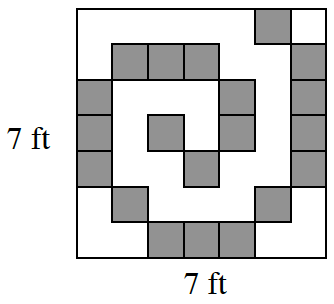### Home > CC1MN > Chapter 2 Unit 2 > Lesson CC1: 2.2.2 > Problem2-34

2-34.Assume that the shaded tiles in the large square at right each have an area of one square foot. Use this information to answer the following questions.

1. What is the total area of the shaded squares?

There are $20$ shaded squares. Make sure you state your answer in terms of area.

2. What is the total area of the un-shaded squares?

There are $29$ un-shaded squares. Make sure you state your answer in terms of area.

3. Find the total number of square feet of area in the figure in two different ways.

You can use addition or multiplication.

Addition would be adding the area of the shaded squares and the area of the un-shaded squares. To use multiplication, what numbers could you multiply? Why does that make sense?